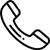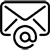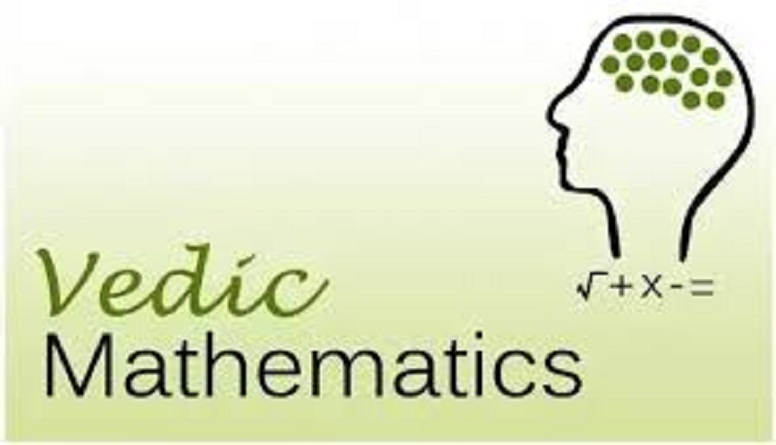+91 9535-191-035Back# Fastest math calculations using Vedic mathematics sutras

Vedic Math

The sutra: URDHVA TIRYAGBHIYAM

Meaning vertically and cross wise

Is the  general formula applicable to all cases of multiplication and in the division of a large number by another large number

To make things more simple using the vertically and crosswise does not require multiplication tables knowledge beyond 5 x5

Let’s solve simple  8 x 7

Steps:

check how far the given numbers are below 10

8      2

7      3

Subtract one number’s deficiency from the other number

8      2

7      3

5

Multiply the deficiencies together

8      2

7      3

5      6

Next Let us solve 7 x 6

7      3

6      4

3      12

Here you find there is a carry over where the one from 12 above goes to add on with 3 to make it 4 as below

7      3

6      4

4      2

Now we will see how to use vertically and crosswise for multiplying numbers close to base 100

Example Multiply 88 x 98

Normally the above problem seems not so easy to solve under conventional math system but it can be solved in no time using Vedic sutra

check how far the given numbers are below 10

Subtract one number’s deficiency from the other number

Multiply the deficiencies together

88    12

98    2

86    24

Ans: 8524

PARAVARTYA YOJAYET

Means TRANSPOSE AND APPLY

Consider the division by divisors of more than 1 digit and when the divisors are slightly greater than the powers of 10

Example Divide 1225 by 12.

Step 1 : (From left to right ) write the Divisor leaving the first digit, write the

other digit or digits using negative (-) sign and place them below the divisor

as shown.

12

-2

‾‾‾‾

Step 2 : Write down the dividend to the right. Set apart the last digit for the

remainder.

12           122     5

– 2

Step 3 : Write the 1st digit below the horizontal line drawn under

the dividend. Multiply the digit by –2, write the product below the 2nd digit

12        122                 5

-2         -2

10

Since 1 x –2 = -2and 2 + (-2) = 0

Step 4 : We get second digits’ sum as ‘0’. Multiply the second digits’ sum

thus obtained by –2 and writes the product under 3rd digit and add.

12        122                 5

-2         -20

102                 5

Step 5 : Continue the process to the last digit.

12        122                 5

-2         -20               -4

102                 1

Step 6: The sum of the last digit is the Remainder and the result to its left is

Quotient.

Thus Q = 102 andR = 1

Courtesy from :http://tutorpixie.com/blog/tag/vedic-sutra-working-examples

AA Academy conducting Vedic Mathematics, online classes and also Abacus Mental Arithmetic Singapore Math, Spelling Bee, Grammar, Phonics, Handwriting, Midbrain Activation